We do not support landscape mode yet.
Please go back to portrait mode for the best experience
Question of The Day22-11-2019

A shopkeeper marked the price of an article in such a way that after allowing the discount of 10%, he gains 15%. If the cost price of article is ? 39960, then what will be the marked price?

Correct Answer : c ) ? 51060

Explanation :

Quick Approach 1:

We know that,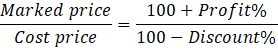So, according to the question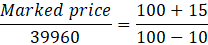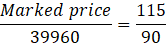MP = ? 51060

Hence, (c) is the correct answer.

Quick Approach 2:

We know that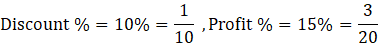Let us assume 10 units corresponds to MP and so, 9 units will correspond to SP

Let us assume 20 units corresponds to CP and so, 23 units will correspond to SP

As SP is same thus equating the ratios to equate the units of SP

Now,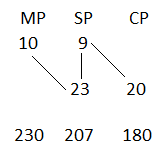From the above figure, we can say that

180 units correspond to ? 39960

1 unit will correspond to ? 222

230 units = 222 × 230 = ? 51060

As 230 units are the value of MP so our MP will be ? 51060

Hence, (c) is the correct answer.

Basic Method:

Let the Market Price (MP) of an article is ? x

Selling Price (SP) = MP – Discount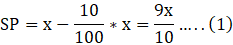Now,

SP = Cost Price (CP) + Profit

From equation (1)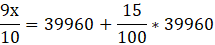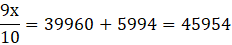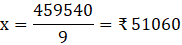Therefore, the marked price of the article is ? 51060.

Hence, (c) is the correct answer.

Questions related to Environment and Ecology are asked in various government exams like SSC CGL,  SSC MTS, SSC CPO, SSC CHSL, RRB JE, RRB NTPC, RRB GROUP D, RRB OFFICER SCALE-I, IBPS PO, IBPS SO,  RRB Office Assistant, IBPS Clerk, RBI Assistant, IBPS RRB OFFICER SCALE 2&3, etc. Try and attempt the Question of the Day to prepare better for your exams.

Current affairs are must to read to prepare the General Awareness section comprehensively. Read Daily Current Affairs here.